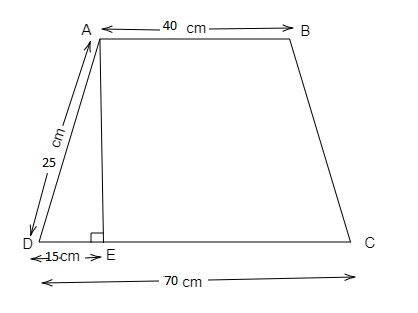# The parallel side of a trapezium are 40 and 70 cm. If the non parallel side are equal, each being 25 cm, find the area of the trapezium

Given:

The parallel sides of a trapezium are 40 cm and 70 cm long. Its non-parallel sides are both equal, each being 25 cm long.

To do:

We have to find the area of the trapezium.

Solution:Height of the trapezium is AE.

$AD^2=AE^2+DE^2$

$25^2=AE^2+15^2$

$625=AE^2+225$

$AE^2=625-225=400$

$AE=\sqrt{400}=20$

We know that,

Area of a trapezium of height h and non-parallel sides a and b$=\frac{1}{2}\times h(a+b)$

$=\frac{1}{2}\times 20 \times (40+70)$

$=10\times(110)$

$=1100\ cm^2$

The area of the trapezium is $1100\ cm^2$.

Updated on: 10-Oct-2022

139 Views